# Fine topology

(diff) ← Older revision | Latest revision (diff) | Newer revision → (diff)

in potential theory

The weakest topology in which all superharmonic functions onare continuous. Objects related to the fine topology are described as "fine" , "finely" , etc.

The notion of fine topology is closely connected with that of a thin set (cf. also Thinness of a set). The fine topology is stronger that the usual Euclidean topology on, i.e. every Euclidean-open set is finely open. A fine neighbourhood of a pointis a set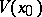such that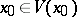and such that the complementis a thin set at. The finely-open sets are unions of pre-images under mappings by superharmonic functions of the extended real lineand of intervals of the form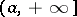,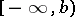,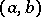,. Every superharmonic function on an open setis finely continuous on. A set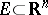is thin at a point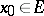if and only ifis a finely-isolated point of.

Letbe a fine-limit point of, that is,is not thin at, and letbe a function defined on. The numberis called the fine limit ofatif for every neighbourhood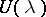ofinthere exists a fine neighbourhoodofsuch that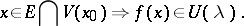Ifis the fine limit ofat, then there exists a fine neighbourhoodsuch thatis an ordinary limit atof the restriction(Cartan's theorem).

Letbe a closed set, thin at a point, and let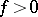be a superharmonic function defined onin a neighbourhood of. Thenhas a fine limitat.

A fine topology has also been constructed in axiomatic potential theory (cf. ).

How to Cite This Entry:
Fine topology. Encyclopedia of Mathematics. URL: http://encyclopediaofmath.org/index.php?title=Fine_topology&oldid=19006
This article was adapted from an original article by E.D. Solomentsev (originator), which appeared in Encyclopedia of Mathematics - ISBN 1402006098. See original article